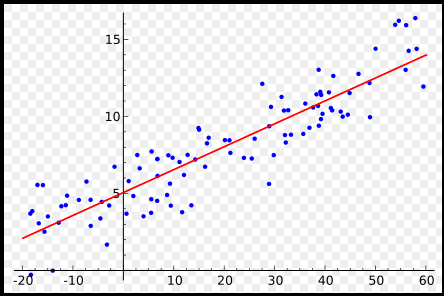## 线性回归

### code

#### data

``````x_train = np.array([[3.3], [4.4], [5.5], [6.71], [6.93], [4.168],
[9.779], [6.182], [7.59], [2.167], [7.042],
[10.791], [5.313], [7.997], [3.1]], dtype=np.float32)

y_train = np.array([[1.7], [2.76], [2.09], [3.19], [1.694], [1.573],
[3.366], [2.596], [2.53], [1.221], [2.827],
[3.465], [1.65], [2.904], [1.3]], dtype=np.float32)
``````

``````x_train = torch.from_numpy(x_train)

y_train = torch.from_numpy(y_train)
``````

#### model

``````class LinearRegression(nn.Module):
def __init__(self):
super(LinearRegression, self).__init__()
self.linear = nn.Linear(1, 1)  # input and output is 1 dimension

def forward(self, x):
out = self.linear(x)
return out
model = LinearRegression()
``````

``````criterion = nn.MSELoss()
optimizer = optim.SGD(model.parameters(), lr=1e-4)
``````

#### train

``````num_epochs = 1000
for epoch in range(num_epochs):
inputs = Variable(x_train)
target = Variable(y_train)

# forward
out = model(inputs) # 前向传播
loss = criterion(out, target) # 计算loss
# backward
loss.backward() # 方向传播
optimizer.step() # 更新参数

if (epoch+1) % 20 == 0:
print('Epoch[{}/{}], loss: {:.6f}'.format(epoch+1,
num_epochs,
loss.data))
``````

``````optimzier.zero_grad()
``````

#### validation

``````model.eval()
predict = model(Variable(x_train))
predict = predict.data.numpy()
``````

ok，在这篇文章中我们使用pytorch实现了简单的线性回归模型，掌握了pytorch的一些基本操作，下一节我们将使用logistic回归对MNIST手写字体数据集做识别。